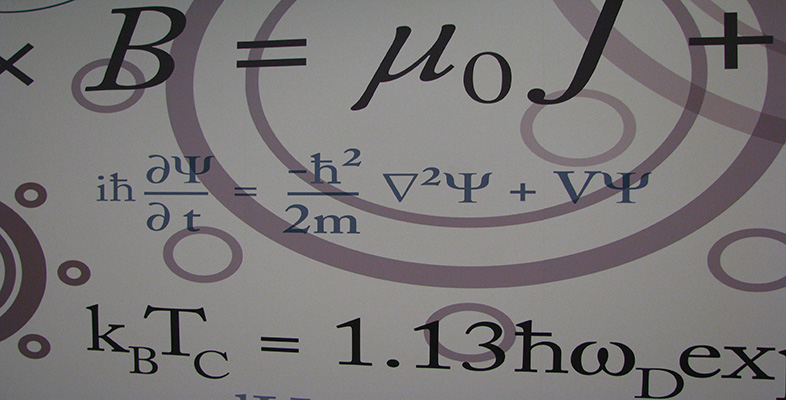Number systems

This free course is available to start right now. Review the full course description and key learning outcomes and create an account and enrol if you want a free statement of participation.

Free course

1.2 Real numbers

The rational and irrational numbers together make up the real numbers. The set of real numbers is denoted by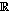. Like rationals, irrational numbers can be represented by decimals, but unlike the decimals for rational numbers, those for irrationals are neither finite nor recurring. All such infinite non-recurring decimals represent real numbers. Each real number can be represented as a point on the number line considered in Section 1.1, which is often known as the real line, and each point on this line represents a real number.

We shall need to use the usual arithmetical operations on real numbers, and we now list the properties which we assume these operations satisfy.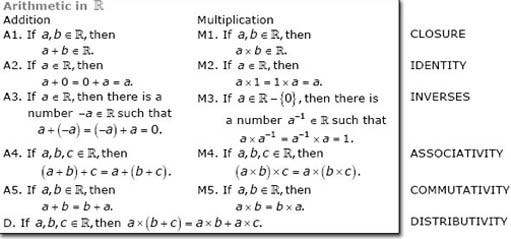In properties A3 and M3 the numbers −a and a−1 are known as the additive inverse (or negative) of a and the multiplicative inverse (or reciprocal) of a, respectively.

The rational numbers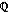also satisfy all the above properties; that is, ifis replaced bythroughout in the box above, then the properties are still true. We shall show later that the same is true for the complex numbers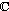and for some sets of numbers in modular arithmetic. However, if we restrict ourselves to the integers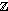, then one of these properties is no longer true.

A set with all these properties is known as a field.

Exercise 2

• (a)  Show by means of a counter-example thatdoes not have property M3.

• (b)  Which integers have a multiplicative inverse in?

Solution

• (a)  There is no integer 2−1 such that 2 × 2−1 = 2−1 × 2 = 1, for example.

• (b)  The numbers 1 and − 1 both have a multiplicative inverse in. In each case the number is its own inverse; such a number is sometimes described as being self-inverse.

In this course we shall be concerned with polynomial equations.

Definition

A polynomial equation in x of degree n is an equation of the form p(x) = 0, where p(x) is a polynomial of degree n.

Polynomial equations (and polynomials) of degrees 1, 2 and 3 are called linear, quadratic and cubic, respectively.

Recall that the formula for the solutions of the quadratic equation ax2 + bx + c = 0 is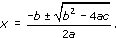This equation is known as the quadratic formula.

Exercise 3

Solve the following quadratic equations, stating how many solutions each equation has in.

• (a)  x2 − 7x + 12 = 0

• (b)  x2 + 6x + 9 = 0

• (c)   2x2 + 5x − 3 = 0

• (d)  2x2 − 2x − 1 = 0

• (e)   x2 − 2x + 5 = 0

Solution

• (a)  This equation has two solutions in.

•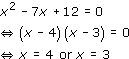• (b)  This equation has one solution in.

•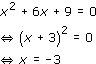• (c)  This equation has two solutions in.

•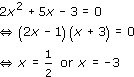• (d)  This equation has two solutions in.

•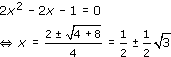• (e)  Since 4 − 20 = −16, which is negative, this equation has no solutions in.

•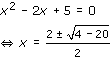We notice from the results of Exercise 3 that some quadratic equations have two real solutions, some have only one and some have none. In either of the first two cases, the solutions may be rational or irrational. Although you may be accustomed to equations with integer coefficients such as those in Exercise 3, these results still apply if some or all of the coefficients are irrational; that is, if the coefficients are any real numbers. Since a quadratic equation with coefficients incan have no solution in, working with the set of real numbers does not enable us to find solutions to all quadratic equations.

We end this section by showing that although a quadratic equation may have no real solutions, this is not true for a cubic equation.

Consider the cubic polynomial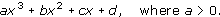For x ≠ 0, the polynomial can be written as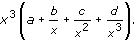Since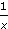,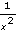and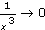as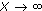, the sign of the polynomial will be positive when x is large and positive, and negative when x is large and negative. Hence the value of the polynomial must change from negative to positive at least once as x increases, so it would appear that it must be zero at one value of x (at least). A similar argument can be used if a < 0. Hence the equation ax3 + bx2 + cx + d = 0, where a ≠ 0, has at least one real solution. This result can be generalised to show that any polynomial equation of degree n, where n is odd, has at least one real solution.

We are assuming here that the graph of a cubic polynomial is an unbroken curve – an assumption we made in OpenLearn course M208_4 Real functions and graphs. This assumption will be justified in the analysis units.

M208_6